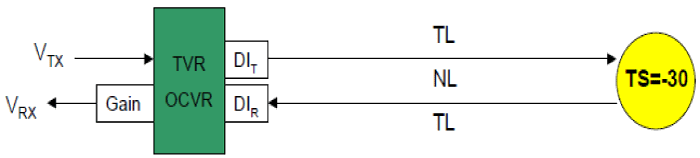Figure 2: Measuring detection range of acoustic instrument. Detection threshold (DT) was computed using the equation below: DT = SL-2 TL+TS+DIT+DIR -NL+OCVR 2 TL ≤ SL-DT+TS+DIT+DIR-NL+ OCVR 2 TL ≤ 220+70-30+30+30-30-178=122 20 log R +α R ≤ 55 R ≤ 180 m Where R is maximum detection range of acoustic instrument, SL is Source Level, TL is Transmission Loss, TS is Target Strength, DIT, DIR is directivity index for transmitting and receiving, respectively, NL is Noise Level; OCVR is open circuit voltage response. For data processing, the acoustic files contain run length packed and coded voltage samples.